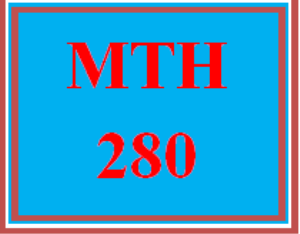# MTH 280 Weekly MyMathLab Week 1 Checkpoint

PLDZ-9125 Free In Stock
\$ 0.00 USD
Description

***************************************************************

~~~~~~~~~~~~~~~~~~~~~~~~~~~~~~~~~~~~~~~~~~~~~~~~~

You can buy more tutorials at a more favorable price from the below link.

***************************************************************

---------------------------------------------------------------------------------------------------------------------

MTH 280 Weekly MyMathLab Week 1 Checkpoint

1.1 Functions and Their Graphs

o       Find the domain and range of functions.

o       Determine if graphs represent functions.

o       Graph and identify symmetries of a function and state where it is increasing and decreasing.

1.2 Combining Functions; Shifting and Scaling Graphs

o       Identify and write equations for functions that involve translations.

o       Shift the graph of a function and write an equation for the shifted graph.

o       Graph functions using transformations.

o       Identify and write equations for functions that involve scaling.

1.3 Trigonometric Functions

o       Use the arc length formula.

o   Evaluate trigonometric functions.

o       Graph and identify characteristics of trigonometric functions.

o   Use the addition formulas for sine and cosine.

o   Use the half-angle formulas for sine and cosine.

o   Solve trigonometric equations.

o   Use the law of sines and the law of cosines.

2.1 Rates of Change and Tangents to Curves

o       Find the average rate of change of a function over an interval.

o   Find the slope of the tangent line at a given point.

2.2 Limit of a Function and Limit Laws

o       Find the limit from graphs.

o   Find the limit of algebraic functions.

o   Find the limit of trigonometric functions.

o   Find the limit using the rules for limits.

o   Evaluate the limit of average rates of change.

o   Use the Sandwich Theorem.

o   Estimate limits.

Recent Reviews Write a Review
0 0 0 0 reviews